Gice

Technology and General BlogIn bash shell, the test command compares one element against another and returns true or false. In bash scripting, the test command is an integral part of the conditional statements that control logic and program flow.

This guide demonstrates how to use the bash test command.

## Test command

The test command takes an EXPRESSION as an argument. After calculating the EXPRESSION, the test returns a value to the bash variable “\$?”. If the value is 0, then the expression evaluation was true. If the value is 1, then the expression evaluation was false.

There are two syntaxes for using the test command.

\$ test EXPRESSION
\$ [ EXPRESSION ]

Note that in the case of “[“, there’s a space at both ends of the EXPRESSION.

## Test usage

Here’s a short example of how the test command works. We’ll be checking whether 1 equals 2. If true, then the output will be “true”. Otherwise, the output will be “false”.

\$ test 1 -eq 2 && echotrue|| echofalse

Let’s break it down.

• test: The test command.
• 1: The first element for comparison.
• -eq: Comparison method (whether values are equal).
• 2: The second element for comparison.

If the test portion is true, then the first echo command will execute. Otherwise, the second echo command will execute.

The same command can be expressed using “[“.

\$ [ 1 -eq 2 ] && echotrue|| echofalse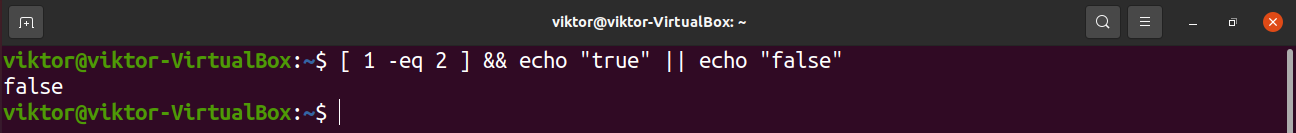## Expression

The expression is what gives the test command its true power. The test can use strings, files, and integers for comparison. Here’s a quick list of all the available test expression formats.

String
In programming, a string is a set of characters that are generally used to represent text. For example, “hello world” in the following echo command is treated as a string.

\$ echo “hello world.”The test command supports the following string expressions.

• -n <string>: String length is non-zero.
• -z <string>: String length is zero.
• <string>: String value is non-zero (quivalent to “-n <string>”).
• <string_a>= <string_b>: Both string_a and string_b are equal.
• <string_a> != <string_b>: The strings string_a and string_b aren’t equal.

Let’s try out these expressions.

\$ [ -n “hello world” ] && echotrue|| echofalse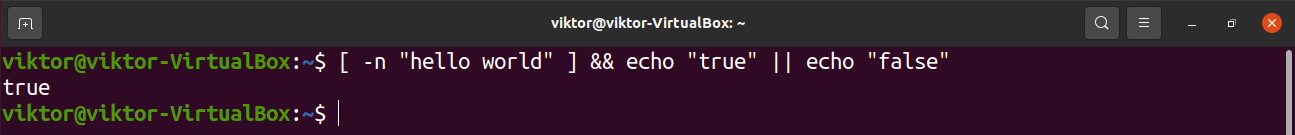\$ [ -z “hello world” ] && echotrue|| echofalse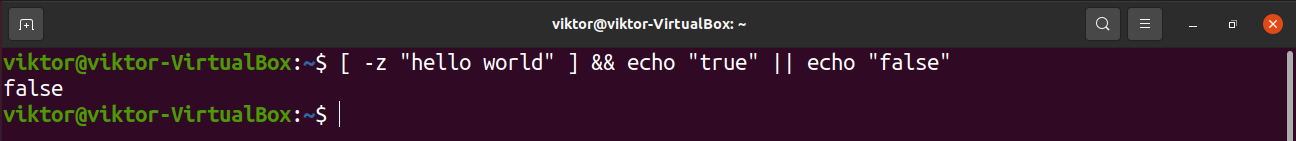\$ [ “hello world” != “Hello World” ] && echotrue|| echofalse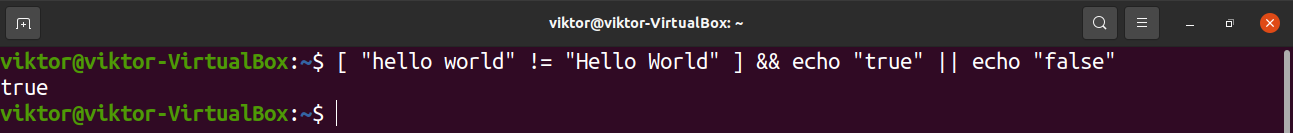\$ [ “hello world” = “Hello World” ] && echotrue|| echofalse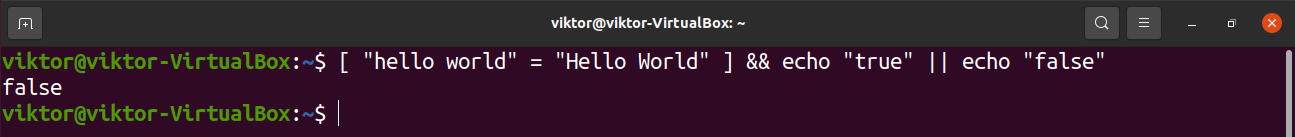Integer

The very first example in this guide demonstrates integer comparison. There are more ways to compare integers.

• <integer_a> -eq <integer_b>: Integer_a is equal to integer_b.
• <integer_a> -ne <integer_b>: Integer_a is not equal to integer_b
• <integer_a> -ge <integer_b>: Integer_a is greater than or equal to integer_b.
• <integer_a> -gt <integer_b>: Integer_a is greater than integer_b.
• <integer_a> -le <integer_b>: Integer_a is less than or equal to integer_b.
• <integer_a> -lt <integer_b>: Integer_a is less than integer_b.

Let’s put these expressions into action.

\$ [ 5 -eq 10 ] && echotrue|| echofalse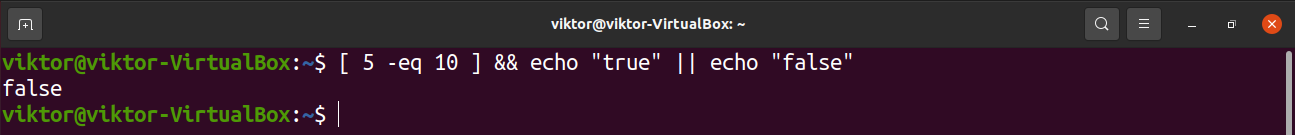\$ [ 5 -gt 2 ] && echotrue|| echofalse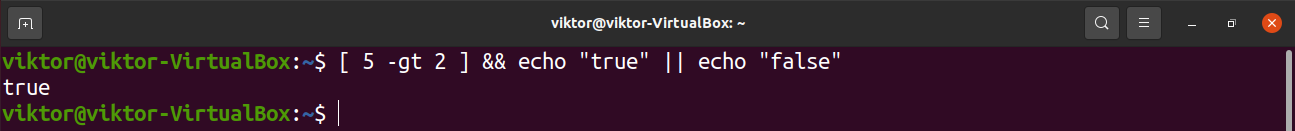\$ [ 4 -le 5 ] && echotrue|| echofalse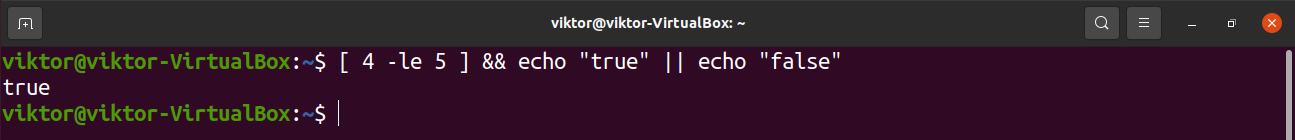File

Files can also be part of the expression. Here’s the list of supported file expressions.

• <file_a> -ef <file_b>: Both file_a and file_b have similar device and inode number. If it’s true, then it signifies that the files are most likely symlinked. Learn more about Linux symbolic links.
• <file_a> -nt <file_b>: In terms of modification date, file_a is newer than file_b.
• <file_a> -ot <file_b>: File_a is older than file_b.

The rest of the supported file expressions are related to a single property of a single file.

• -e <file_a>: File_a exists.
• -f <file_a>: File_a exists and a regular file.
• -d <file_a>: File_a exists and is a directory.
• -r <file_a>: File_a exists with read permissions.
• -w <file_a>: File_a exists with write permissions.
• -x <file_a>: File_a exists with execute permissions.
• -s <file_a>: File_a exists and file size is greater than zero.
• -O <file_a>: File_a exists and the owner is effective user ID.
• -G <file_a>: File_a exists and the owner is effective group ID.
• -h <file_a>: File_a exists and it’s a symbolic link.
• -L <file_a>: File_a exists and it’s a symbolic link.
• -b <file_a>: File_a exists. It’s a block-special file.
• -c <file_a>: File_a exists. It’s a character-special file.
• -S <file_a>: File_a exists. It’s a socket.

Let’s have a look at some examples.

\$ [ -x /usr/bin/bash ] && echo \$?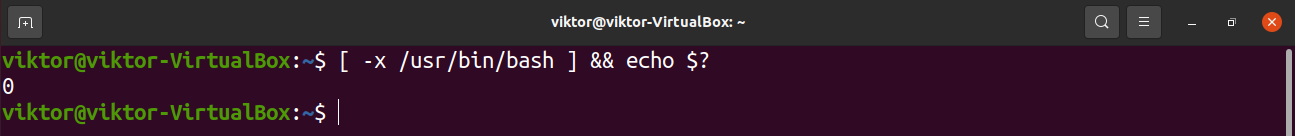\$ [ -s /bin/bash ] && echo \$?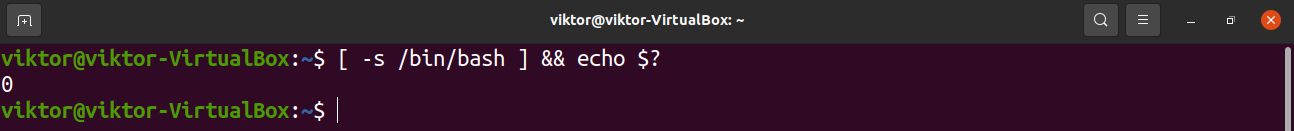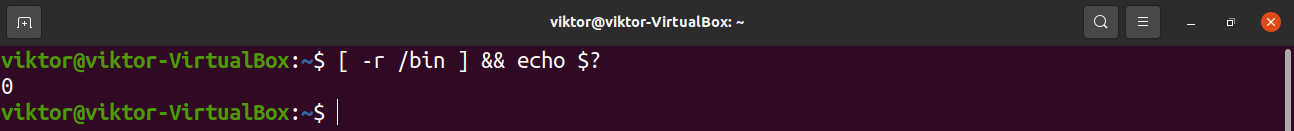\$ [ -e /hello_world ] && echo “true” || echo “false”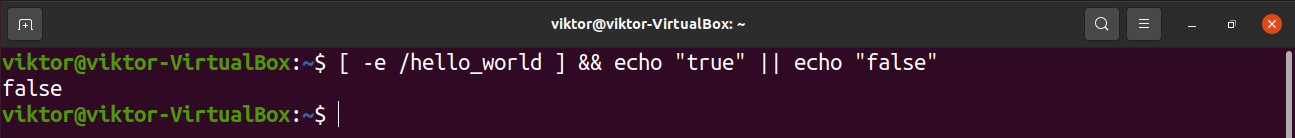## Implementing test in bash scripts

So far, we’ve demonstrated how to use the test command to determine whether a certain condition is true or false. We can implement this into bash scripts to make useful decisions.

Have a look at the following short script.

#!/bin/bash
if [ \$(whoami) = root ]; then
echo “root”
else
echo “not root”
fi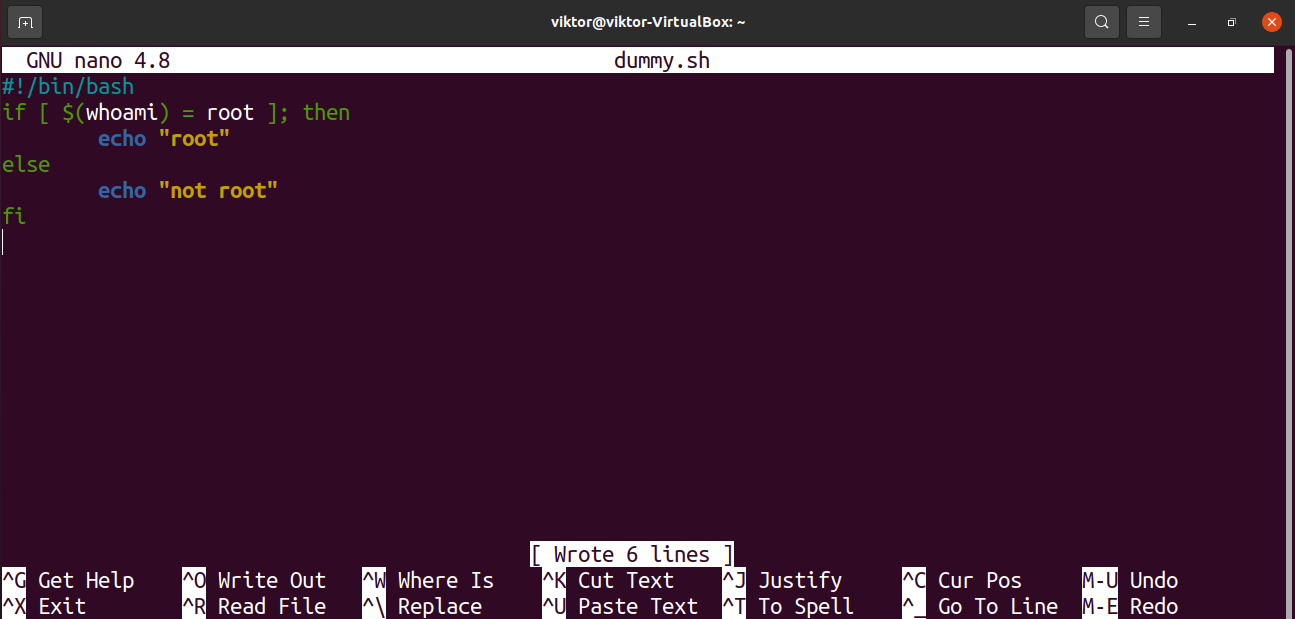Here, the if statement will check whether the condition is true or false. Using the test command, we can easily get the Boolean value.

Run the script with and without root privilege.

\$ ./dummy.sh
\$ sudo ./dummy.sh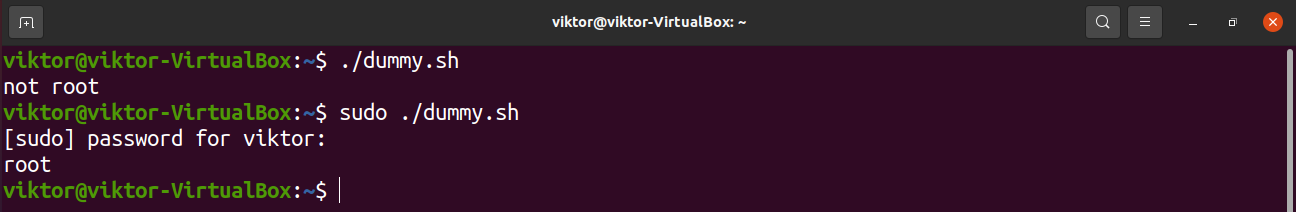It’s a simple if-else statement demonstration. Feel free to check out bash if-else statements for further in-depth applications.

## Final thoughts

The test command is simple but powerful. This guide explains and demonstrates various ways of using the test. The complete list of all the supported expressions is available on the man page.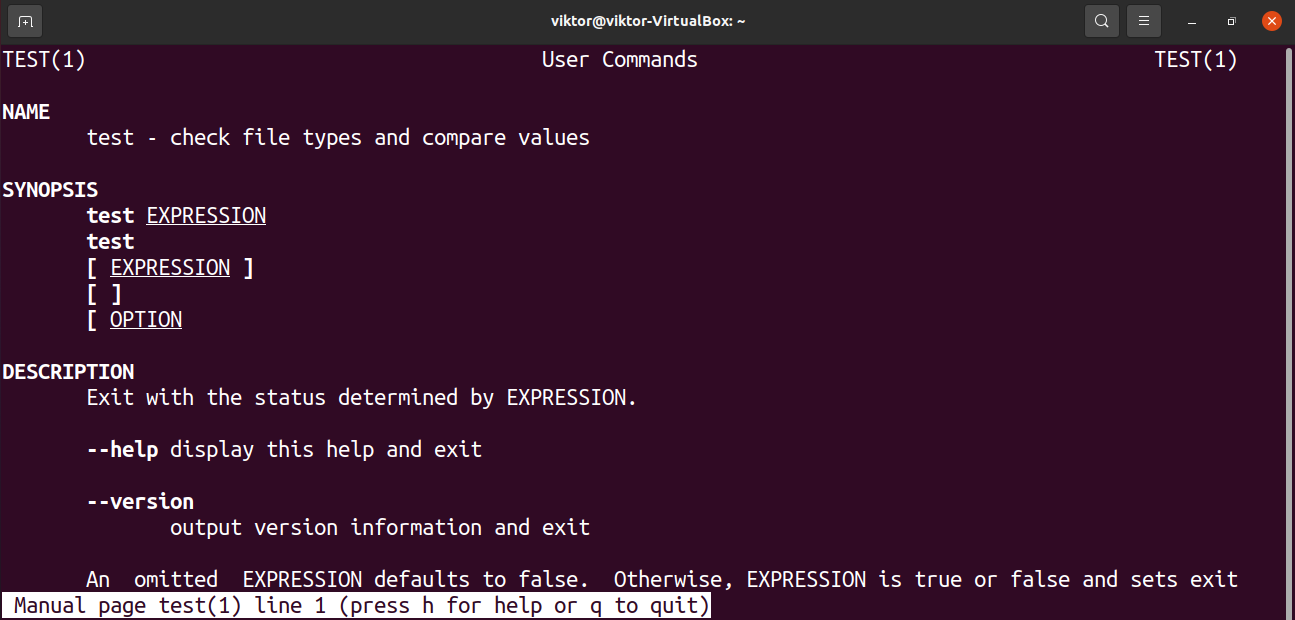Happy computing!